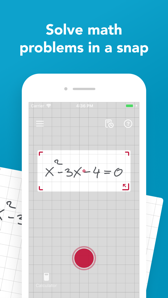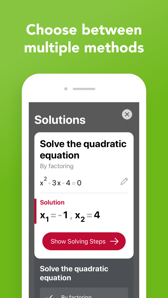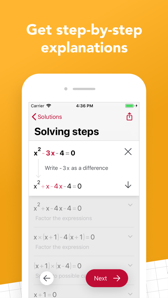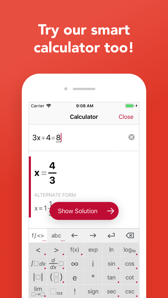Photomath

iPhoneAPP ID

919087726

6.1.0

••••Learn math, check homework and study for upcoming tests and ACTs/SATs with the most used math education app on the planet. Photomath is FREE and works without wi-fi or data. Whether you’re on the bus, in a cave or just offline, core Photomath is always available, because data matters.

From basic arithmetic to advanced calculus and geometry, learn with Photomath. Understand core concepts to help you advance in math and feel confident in class!

KEY FEATURES

● No internet or data required

● Free to use

● Step-by-step explanations for every solution

● Exclusive how-to animations

● Scroll through multiple solving methods per problem

● Multi-functional scientific calculator

● Interactive graphs

MATH TOPICS COVERED

● Basic Math/Pre-Algebra: arithmetic, integers, fractions, decimal numbers, powers, roots, factors

● Algebra: linear equations/inequalities, quadratic equations, systems of equations, logarithms, functions, matrices, graphing, polynomials

● Trigonometry/Precalculus: identities, conic sections, vectors, matrices, complex numbers, sequences and series, logarithmic functions

● Calculus: limits, derivatives, integrals, curve sketching

● Statistics: combinations, factorials

Featured in Huffington Post, Forbes, TIME, CNN, EdSurge, Guiding Tech, The Verge, TechCrunch and more.

Suggestions or questions? Email us at support@photomath.net

Website: www.photomath.app

What's new in 6.1.0:

• Now you can use the flashlight to scan math problems

• Edit problems from the history list

• Bug fixes

• 开发商：Photomath, Inc.
• 类别：Education